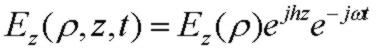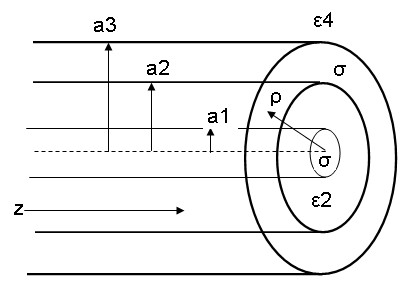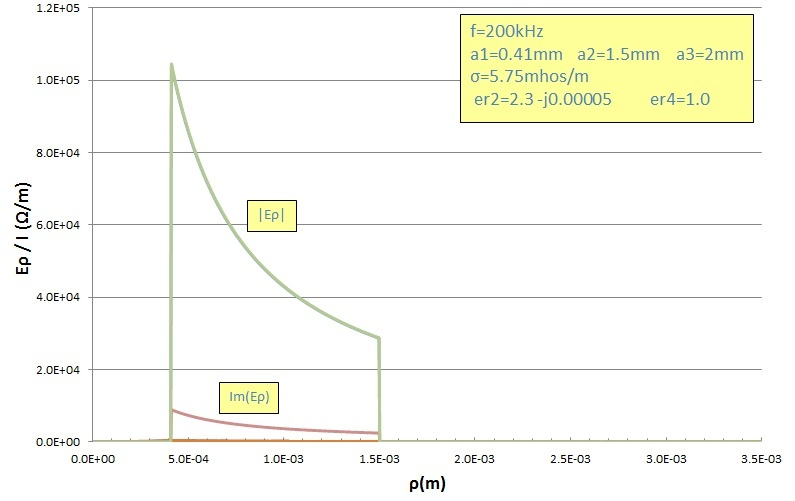Coaxial Cable 200 kHz Reference Data

Jan 03, 2013

The graphs and data below show results of accurate calculations of the propagation constant h, characteristic impedance Zo, distributed series impedance Z and longitudinal (axial) electric field Ez(ρ), azimuthal magnetic field Hφ(ρ) and radial electric field Eρ(ρ) for the circular symmetric principal TM propagation mode for typical RG58U coaxial cable at a low frequency of 200kHz. At lower frequencies the characteric impedance becomes strongly complex and deviates dramatically from the nominal high-frequency value. In addition, fields penetrate outside the outer conductor layer of the coaxial cable and can lead to interference and crosstalk with external lines. The graphs are accurate to within the plot resolution and the numerical data is accurate to within digit of the least significant digit displayed. The sign convention and definition of propagation constant h is that of Stratton:The geometry and symbols used are shown below:The conductivity σ = 5.75E7 mhos/m of the inner and outer conductors are the same. The dielectric constants of the inner and outer dielectrics are ε2 = (2.3, -0.00005) and ε4= (1.0, 0) respectively. The radii of the conductors are a1 = 0.41mm, a2 = 1.5mm and a3 = 2.0 mm. The permitivity of free space is taken as εo = 8.854 pF/m.

The Calculation

The propagation constant h and characteristic impedance Zo were computed using the formalism presented by Stratton (1941). The fields were matched at the 3 interfaces and the roots of the transcendental equation for the h were calculated numerically to high precision.

Propagation Constant and Characteristic Impedance

The computed values for the propagation constant h, characteristic impedance Zo and distributed line impedance Z are:
```
h:   6.9107303e-3  +   j5.9192989e-4   m-1         attenuation: 5.1415e-3 dB/m
Zo:  55.747939     +   j4.773799  Ω
Z:   6.59893e-2    -   j3.82433e-1  Ω/m
```
For comparison, the results WITH AN INFINITE OUTER CONDUCTOR are:
```
h:   6.9105789e-3  +   j5.9159116e-4   m-1         attenuation: 5.1386e-3 dB/m
Zo:  55.746717     +   j4.771066  Ω
Z:   6.59501e-2    -   j3.82420e-1 Ω/m
```
At 200 kHz (and higher frequencies), the results for the finite thickness outer conductor are almost identical to the result with an infinite outer conductor. The imaginary part of Zo (keeping in mind Stratton's sign convention above) indicates that Zo has a small capacitive component. The imaginary part of Z indicates that the distributed series impedance is mainly inductive at this frequency.

Fields

The fields are shown below demonstrating the penetration outside the outer conductor of the coaxial cable. The values plotted are field values divided by the total current in the inner conductor (which is at 200 kHz almost identical to the return current in the outer conductor as discussed in Daywitt (1991)). The real and imaginary parts as well as the field magnitudes are all displayed. The radial electric field Eρ(ρ) includes a magnified view showing the field penetration beyond the outer conductor. By comparison with the values for the axial electric field Ez(ρ), it is seen that the electric field penetrating outside the outer conductor is almost exactly radial :Reference:
• Coaxial Cable Characteristic Impedance vs Frequency
• The Electromagnetic Theory of Coaxial Transmission Lines, S. Schelkunoff, 1934, Bell System Technical Journal, p. 532
• Exact Principal Mode Field for a Lossy Coaxial Line, W. C. Daywitt, IEEE Trans. MTT, v39, #8, 1991 p. 1313
• Electromagnetic Theory, J. Stratton, 1941, McGraw Hill, pp. 551-554
• Electrodynamics, A. Sommerfeld, Lectures on Theoretical Physics, VIII Academic Press, 1952.
• Fields and Waves in Communication Electronics, S. Ramo, J. Whinnery and T. Van Duzer, 2nd Edn. 1984, John Wiley & Sons, pp. 249-252
• Inductance Calculations, F. Grover, 1946, 1973, Dover, Instrument Society of America, p. 42, pp.262 - 282.
• Waveguide Handbook, N. Marcuvitz, 1986, Peter Peregrinus Ltd. pp. 17-25, p. 72